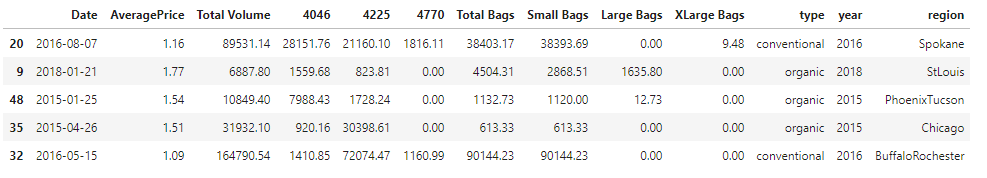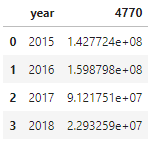# PyJanitor and Pandas Method Chaining

I want to share a Python package that makes your pandas code more readable.

## How do I like my pandas code

When I write code in `pandas` to make a dataframe, I like to write with method chaining.

For example, I might write:

``````import pandas as pd
df = (
raw_data
.assign(order_date = lambda _df: pd.to_datetime(_df['order_date']))
[lambda _df: _df['order_date'] >= pd.to_datetime('2019-11-09')]
.groupby(['category'], axis=1)
.agg({
'dollars': 'sum',
'volume': 'sum
})
)
``````

``````import pandas as pd
raw_data['order_date'] = pd.to_datetime(raw_data['order_date'])
filtered = raw_data[raw_data['order_date'] >= pd.to_datetime('2019-11-09')]
grouped = df.groupby(['category'], axis=1)
df = grouped.agg({
'dollars': 'sum',
'volume': 'sum
})
``````

If you come from an R background, you may have recognized the method chaining style from tidyverse piping in R with the `%>%` operator.

### Why do I like my code this way?

Even though both returns the same thing, in my opinion, method chaining is much more readable.

Why? Because it is easier for me to track the “data flow” when I view data processing as a directed acyclic graph (DAG):If I can group all the code for `df1` together and all the code for `df2` together, then I can understand where I can stop worrying about `df1` or `df2`, when a new dataset come in, etc.

In other words, I don’t have to mentally track which variable is pointing to which dataset anymore, especially when a single script pulls in several data sources.

Let me give you an imaginary example

#### Code 1

``````df1 = do_stuff(df)
df2 = do_more_stuff(df1)

another_df1 = do_good_stuff(another_df)
another_df2 = do_more_good_stuff(another_df1)

joined = df2.merge(another_df2, on=...)
``````

#### Code 2

``````df2 = (
df
.do_stuff()
.do_more_stuff()
)

another_df2 = (
another_df
.do_good_stuff()
.do_more_good_stuff()
)

joined = df2.merge(another_df2, on=...)
``````

I think Code 2 is much more readable.

### But my function is not a pandas method?

But how can I make a method called `do_stuff()` for pandas?

Many of my functions are specific to the job at hand, say

``````
def get_sales_in_thanksgiving_week(df):
# implementation here
# ...
return output
``````

and they are usually called with

``````thanksgiving_sales = get_sales_in_thanksgiving_week(df)
``````

which breaks the nice method chaining DAG. How can I apply method chaining in this case?

## Comes PyJanitor

The package `pyjanitor` adds to `pandas` many utility method chaining functions. And it allows you to add custom methods to any pandas dataframes.

## An example

The example I use is the Avocado Prices dataset on Kaggle. You can also read the notebook here.

``````import pandas as pd
``````### Use janitor.then() to chain a function

For example, to get the yearly sales by product ID (PID), I might write a function like this:

``````def get_yearly_sum_by_PID(df, PID):
output = (
df
[['year', str(PID)]]
.groupby(['year'], as_index=False)
.agg({
str(PID): 'sum'
})
.sort_values('year')
)
return output
``````

and to call it as a method, I can use `pyjanitor.then()` to register and `functools.partial()` to supply the parameters:

``````from janitor import then
from functools import partial

df_by_pid = (
.then(partial(get_yearly_sum_by_PID, PID=4770))
)
``````The current design of `janitor.then()` only takes in functions with a single parameter: `df`, so I have to use `functools.partial()` if my function requires extra parameters.

### Other handy functions

PyJanitor has other functions that may help you simplify code that you can find in the documentation. For example,

``````drop_cols = ['Small Bags', 'Large Bags', 'XLarge Bags']
# pandas style
# pyjanitor style
df_no_bags.equals(df_no_bags_pd)
``````
``````df_dt = raw_avocados.to_datetime('Date')
It turns out that the `.then()` method is a simple wrapper using the package `pandas-flavor`:
So, if you don’t want to use `pyjanitor`’s `then()` method to register your own method, an alternative is to follow the example in here.